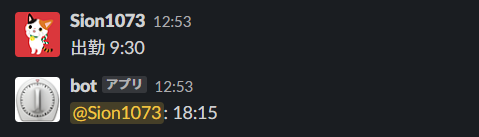# [PYTHON] I started to work at different times, so I made a bot that tells me the time to leave

Because of the recent infectious disease, staggered commuting has started. I made a bot that tells me the time to leave when I post the time to work.

• python 3.6.6
• Heroku

## reference

For the directory structure of the SlackBot project and the cooperation with Heroku, I referred to the following site. https://qiita.com/akabei/items/ec5179794f9e4e1df203

## Source code

I was able to confirm the operation of SlackBot based on the site I referred to above (I closed the fortune), so I will create a code to calculate the leaving time.

・ Calculate the leaving time using the posting time as the arrival time

``````from datetime import datetime, timedelta, timezone
from slackbot.bot import listen_to

@listen_to(r'^Attendance\$')
def work_time(message):
JST = timezone(timedelta(hours=+9), 'JST')

start_now = datetime.now(JST)
end_time = start_now + timedelta(hours=8, minutes=45)
``````

Execution result ↓ ↓If this is the case, you will have to send a message immediately after coming to work, so create a pattern that allows you to specify the time.

・ Specify the time of arrival and calculate the time of departure

``````import re
from datetime import datetime, timedelta, timezone

from slackbot.bot import respond_to, listen_to

@listen_to(r'^Attendance\s[0-9]+:[0-9]+\$')
def work_time(message):

JST = timezone(timedelta(hours=+9), 'JST')
current = datetime.now(JST)

text = message.body['text']
result = re.match(".*\s([0-9]+):([0-9]+)", text)

hour = result.group(1)
minute = result.group(2)

start_now = datetime(
year=current.year,
month=current.month,
day=current.day,
hour=int(hour),
minute=int(minute))
end_time = start_now + timedelta(hours=8, minutes=45)
Execution result ↓ ↓